Function Repository Resource:

# ImplicitCurvature

Compute the implicit curvature of a curve

Contributed by: Wolfram Staff (original content by Alfred Gray)
 ResourceFunction["ImplicitCurvature"][c,{x,y}] computes the implicit curvature of a plane curve c with respect to variables x and y.

## Details and Options

An implicit curve is a planar curve written using only two coordinate variables, such as x and y.

## Examples

### Basic Examples (2)

Get the equation for a bow curve:

 In:=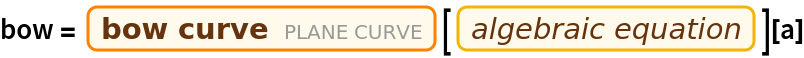Out=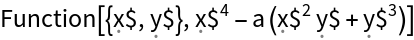Compute the implicit curvature:

 In:=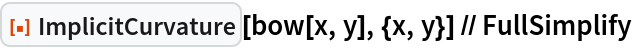Out=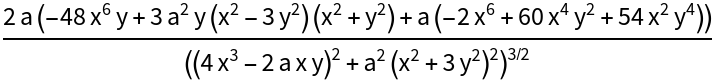Get the equation for a cissoid of Diocles:

 In:=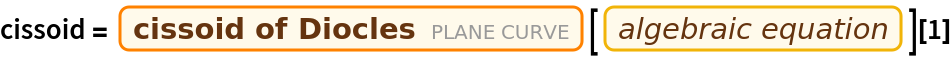Out=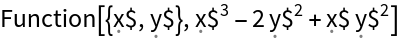Compute the implicit curvature:

 In:=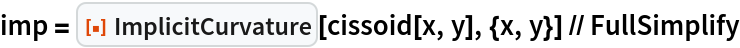Out=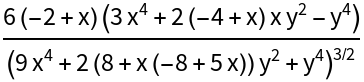Plot the cissoid (orange) and intrinsic curvature (yellow); the background is for values other than zero:

 In:=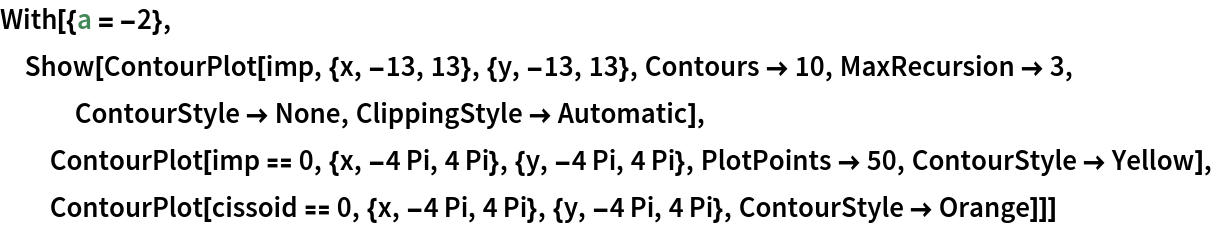Out=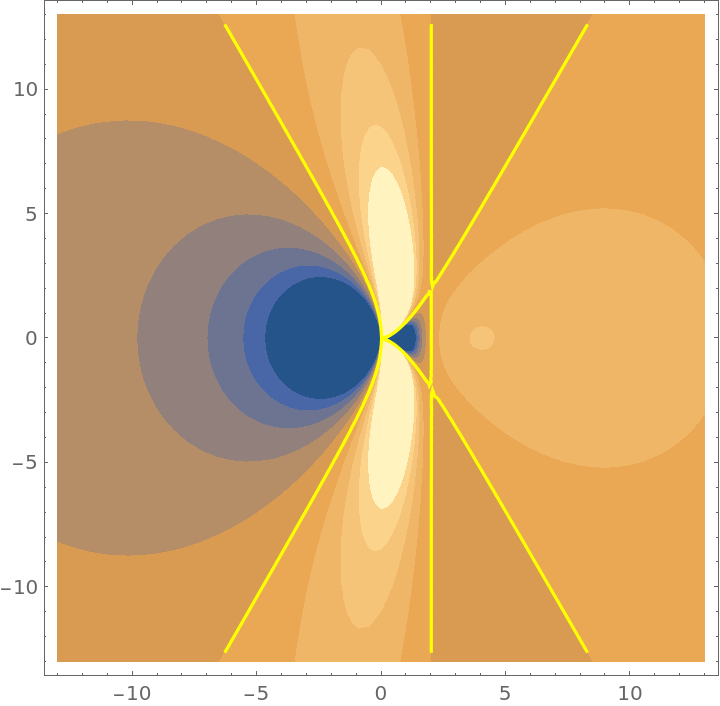Enrique Zeleny

## Version History

• 1.0.0 – 21 July 2020